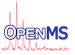OpenMS
ClusterAnalyzer Class Reference

Bundles analyzing tools for a clustering (given as sequence of BinaryTreeNode's) More...

#include <OpenMS/COMPARISON/CLUSTERING/ClusterAnalyzer.h>

## Public Member Functions

ClusterAnalyzer ()
default constructor More...

ClusterAnalyzer (const ClusterAnalyzer &source)
copy constructor More...

virtual ~ClusterAnalyzer ()
destructor More...

std::vector< float > averageSilhouetteWidth (const std::vector< BinaryTreeNode > &tree, const DistanceMatrix< float > &original)
Method to calculate the average silhouette widths for a clustering. More...

std::vector< float > dunnIndices (const std::vector< BinaryTreeNode > &tree, const DistanceMatrix< float > &original, const bool tree_from_singlelinkage=false)
Method to calculate Dunns indices for a clustering. More...

std::vector< float > cohesion (const std::vector< std::vector< Size > > &clusters, const DistanceMatrix< float > &original)
Method to calculate the cohesions of a certain partition. More...

float averagePopulationAberration (Size cluster_quantity, std::vector< BinaryTreeNode > &tree)
Method to calculate the average aberration from average population in partition resulting from a certain step in clustering. More...

void cut (const Size cluster_quantity, const std::vector< BinaryTreeNode > &tree, std::vector< std::vector< Size > > &clusters)
Method to calculate a partition resulting from a certain step in clustering given by the number of clusters. More...

void cut (const Size cluster_quantity, const std::vector< BinaryTreeNode > &tree, std::vector< std::vector< BinaryTreeNode > > &subtrees)
Method to calculate subtrees from a given tree resulting from a certain step in clustering given by the number of clusters. More...

String newickTree (const std::vector< BinaryTreeNode > &tree, const bool include_distance=false)
Returns the hierarchy described by a clustering tree as Newick-String. More...

## Private Member Functions

ClusterAnalyzeroperator= (const ClusterAnalyzer &source)
assignment operator More...

## Detailed Description

Bundles analyzing tools for a clustering (given as sequence of BinaryTreeNode's)

## ◆ ClusterAnalyzer() [1/2]

 ClusterAnalyzer ( )

default constructor

## ◆ ClusterAnalyzer() [2/2]

 ClusterAnalyzer ( const ClusterAnalyzer & source )

copy constructor

## ◆ ~ClusterAnalyzer()

 virtual ~ClusterAnalyzer ( )
virtual

destructor

## ◆ averagePopulationAberration()

 float averagePopulationAberration ( Size cluster_quantity, std::vector< BinaryTreeNode > & tree )

Method to calculate the average aberration from average population in partition resulting from a certain step in clustering.

Parameters
 cluster_quantity desired partition Size analogue to ClusterAnalyzer::cut tree vector of BinaryTreeNode's representing the clustering
Exceptions
 invalid_parameter if desired clustering is invalid
Returns
the average aberration from the average cluster population (number of elements/cluster_quantity) at cluster_quantity
BinaryTreeNode

## ◆ averageSilhouetteWidth()

 std::vector averageSilhouetteWidth ( const std::vector< BinaryTreeNode > & tree, const DistanceMatrix< float > & original )

Method to calculate the average silhouette widths for a clustering.

Parameters
 tree vector of BinaryTreeNode's representing the clustering original DistanceMatrix for all clustered elements started from
Returns
a vector filled with the average silhouette widths for each cluster step

The average silhouette width will be calculated for each clustering step beginning with the first step(n-1 cluster) ending with the last (1 cluster, average silhouette width is 0 by definition).

BinaryTreeNode

## ◆ cohesion()

 std::vector cohesion ( const std::vector< std::vector< Size > > & clusters, const DistanceMatrix< float > & original )

Method to calculate the cohesions of a certain partition.

Parameters
 clusters vector of vectors holding the clusters (with indices to the actual elements) original DistanceMatrix for all clustered elements started from
Returns
a vector that holds the cohesions of each cluster given with clusters (order corresponds to clusters)

## ◆ cut() [1/2]

 void cut ( const Size cluster_quantity, const std::vector< BinaryTreeNode > & tree, std::vector< std::vector< BinaryTreeNode > > & subtrees )

Method to calculate subtrees from a given tree resulting from a certain step in clustering given by the number of clusters.

Parameters
 cluster_quantity Size giving the number of clusters (i.e. starting elements - cluster_quantity = cluster step) tree vector of BinaryTreeNode's representing the clustering subtrees vector of trees holding the trees, tree is composed of cut at given size
Exceptions
 invalid_parameter if desired clusterstep is invalid
BinaryTreeNode

after call of this method the argument clusters is filled corresponding to the given cluster_quantity with the indices of the elements clustered

## ◆ cut() [2/2]

 void cut ( const Size cluster_quantity, const std::vector< BinaryTreeNode > & tree, std::vector< std::vector< Size > > & clusters )

Method to calculate a partition resulting from a certain step in clustering given by the number of clusters.

If you want to fetch all clusters which were created with a threshold, you simply count the number of tree-nodes which are not -1, and subtract that from the number of leaves, to get the number of clusters formed , i.e. cluster_quantity = data.size() - real_leaf_count;

Parameters
 cluster_quantity Size giving the number of clusters (i.e. starting elements - cluster_quantity = cluster step) tree vector of BinaryTreeNode's representing the clustering clusters vector of vectors holding the clusters (with indices to the actual elements)
Exceptions
 invalid_parameter if desired clusterstep is invalid
BinaryTreeNode

after call of this method the argument clusters is filled corresponding to the given cluster_quantity with the indices of the elements clustered

Referenced by SpectraMerger::mergeSpectraPrecursors().

## ◆ dunnIndices()

 std::vector dunnIndices ( const std::vector< BinaryTreeNode > & tree, const DistanceMatrix< float > & original, const bool tree_from_singlelinkage = false )

Method to calculate Dunns indices for a clustering.

Parameters
 tree vector of BinaryTreeNode's representing the clustering original DistanceMatrix for all clustered elements started from tree_from_singlelinkage true if tree was created by SingleLinkage, i.e. the distances are the minimal distances in increasing order and can be used to speed up the calculation
BinaryTreeNode

## ◆ newickTree()

 String newickTree ( const std::vector< BinaryTreeNode > & tree, const bool include_distance = false )

Returns the hierarchy described by a clustering tree as Newick-String.

Parameters
 tree vector of BinaryTreeNode's representing the clustering include_distance bool value indicating whether the distance shall be included to the string